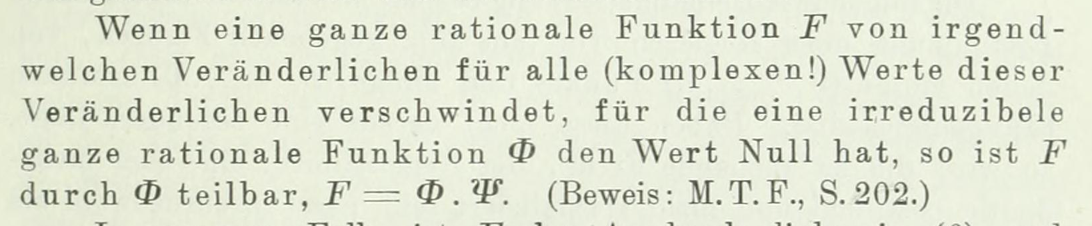# History of Study's Lemma?

The following theorem is usually attributed to Eduard Study:

Let $$f(x,y)$$ and $$g(x,y)$$ be polynomials in two variables over a field, with $$f$$ irreducible. If $$f\nmid g$$ then the curves $$C_f:f=0$$ and $$C_g:g=0$$ have finitely many points of intersection. Consequently, If the field is algebraically closed and $$C_f\subseteq C_g$$ (hence $$C_f\cap C_g$$ has infinitely many points) then $$f|g$$.

However, I have not been able to track down any reference to this result outside of modern textbooks.

Questions:

• What is the original reference for this result?
• What did Study actually prove?
• What was the context?
• Did this result directly influence later versions of the Nullstellensatz?

Thanks.

Edit: I see that Study wrote a book on on the Theory of Ternary Forms (1889). I suppose the result must be in there somewhere.

I found the lemma on page 63 of Study's Einleitung in die Theorie der Invarianten linearer Transformationen auf Grund der Vektorenrechnung (1923).The source cited for the proof is page 202 of Study's Methoden zur Theorie der ternären Formen (1889), where it appears as an endnote and refers to a "less elementary" proof by

O. Hölder, Zum Invariantenbegriff, Math. Naturwiss. Mitteilungen 1, 59–65 (1884).

Volume 1 of the journal is not online, but there is a review at zbMath [thanks, @spin] that explicitly mentions the lemma:

Sind die ganzen rationalen Functionen $$F(x_1,x_2,\ldots,x_m)$$, $$G(x_1,x_2,\ldots,x_m)$$ so beschaffen, dass für alle (reellen oder complexen) Wertsysteme $$x_1,x_2,x_m$$, wofür $$G=0$$ ist, auch die Gleichung $$F=0$$ gilt, und ist G irreduzibel, so ist $$G$$ ein Teiler von $$F$$.
Let the entire rational functions $$F(x_1,x_2,\ldots,x_m)$$ and $$G(x_1,x_2,\ldots,x_m)$$ be such, that for any (real or complex) values $$x_1,x_2,\ldots x_m$$ where $$G=0$$ also $$F=0$$, and let $$G$$ be irreducible, then $$G$$ divides $$F$$.

It's too late (and not productive) to change common practice, but priority wise I would conclude that Study's lemma is Hölder's lemma.

• Review of Hölder's paper "Zum Invariantenbegriff": link – spin Oct 21 at 16:21